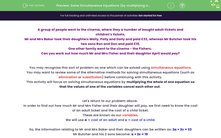# Solve Simultaneous Equations (by multiplying one equation)

In this worksheet, students will learn how to solve simultaneous equations using the elimination method, in which one equation needs to be multiplied. They will also solve contextualised question based on simultaneous equations.Key stage:  KS 4

Year:  GCSE

GCSE Subjects:   Maths

GCSE Boards:   Pearson Edexcel, OCR, Eduqas, AQA,

Curriculum topic:   Algebra

Curriculum subtopic:   Solving Equations and Inequalities Algebraic Equations

Difficulty level:#### Worksheet Overview

A group of people went to the cinema, where they a number of bought adult tickets and children's tickets.

Mr and Mrs Baker took their daughters Molly, Polly and Dolly and paid £33, whereas Mr Butcher took his two sons Ron and Don and paid £19.

One other family went to the cinema - the Fishers.

Can you work out how much Mr and Mrs Fisher and their daughter April would pay?

You may recognise this sort of problem as one which can be solved using simultaneous equations.

You may want to review some of the alternative methods for solving simultaneous equations (such as elimination or substitution) before continuing with this activity.

This activity will focus on solving simultaneous equations by multiplying the whole of one equation so that the values of one of the variables cancel each other out

In order to find out how much Mr and Mrs Fisher and their daughter will pay, we first need to know the cost of an adult ticket and the cost of a child ticket.

These are known as our variables.

We will use a = cost of an adult and c = cost of a child.

So, the information relating to Mr and Mrs Baker and their daughters can be written as: 2a + 3c = 33

Mr Butcher and his 2 sons become: a + 2c = 19

We now have our two equations, and we need to solve them simultaneously:

2a + 3c = 33   (1)

a + 2c  =  19   (2)

At this point with other pairs of simultaneous equations, we would either add or subtract our equations to eliminate one of the variables.

However, that will not help us here since neither the coefficients of a nor of c are the same.

So what we have to do is match one coefficient by multiplying the whole of one equation.

So, if we take equation (2) and multiply it by 2 we get: 2a + 4c = 38

This means that 2 adults and 4 children would pay £38.

We can call this equation (3) and now place it above equation (1):

2a + 4c = 38   (3)

2a + 3c  =  33   (1)

Now we have the same number of a's in both equations, and the signs in front are the same, so we can subtract (1) from (3):

2a + 4c = 38   (3)

2a + 3c  =  33   (1)

c = 5   (3) - (1)

So, a child's ticket costs £5.

Now, we need to find the cost of an adult ticket, by substituting c = 5 into any of our three equations:

a + 2 x 5 = 19

a + 10 = 19

a = 10.

So an adult ticket costs £10.

Now we can find the cost for the Fisher family (which is two adults and one child):

2 x 9 + 5 = 23, so they would pay £23.

If we compare what we have done here with other simultaneous equation pairs, you will see that we have just added in one extra stage where we multiply one equation by a number, in order to equate the coefficients of one variable.

Other than that, everything else is the same process which you may have practised before.

Now, let's look at another pair of simultaneous equations.

This time we have been provided with just the equations without the real-life problem.

Solve 3x + 2y = 10  (1)

and 7x - y = 29    (2)

Notice that the coefficients of x are different, as are the coefficients of y.

But if we double equation (2), we will have 2y in each equation:

3x + 2y = 10  (1)

7x - y = 29    (2) ×2

14x - 2y = 58  (3)

Since the signs in front of the y's are different, we need to add equations (1) and (3):

3x + 2y = 10  (1)

14x - 2y = 58  (3)

17x = 68

x = 4   [divide by 17]

3 × 4 + 2y = 10  [sub into (1)]

12 + 2y = 10

2y = -2  [subtract 12]

y = -1   [divide by 2]

7 x 4 - -1 = 29  [check in (2)]

28 + 1 = 29 [correct]

So x = 4 and y = -1.

Now you should be ready to try solving some pairs of your own!

This process has many steps and can get complicated, so be sure to have a pen and paper handy to help you.

Remember, that we just need to follow our regular process for solving simultaneous equations with one extra step added - we need to multiply the whole of one of the equations so that we can match one of the coefficients so they cancel each other out.

### What is EdPlace?

We're your National Curriculum aligned online education content provider helping each child succeed in English, maths and science from year 1 to GCSE. With an EdPlace account you’ll be able to track and measure progress, helping each child achieve their best. We build confidence and attainment by personalising each child’s learning at a level that suits them.

Get started